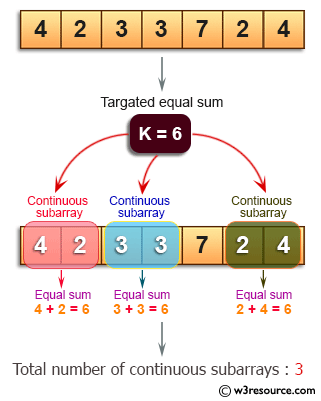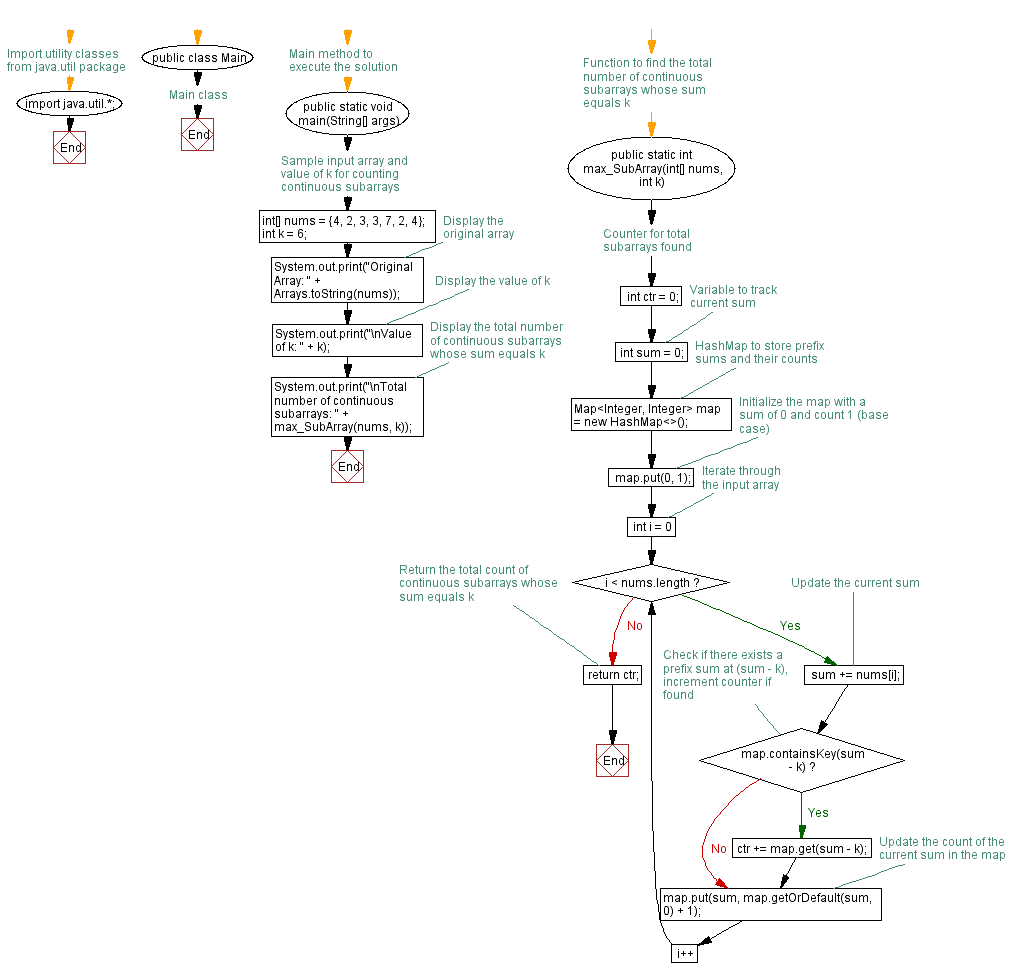# Java Exercises: Find the total number of continuous subarrays in a specified array of integers

## Java Basic: Exercise-202 with Solution

Write a Java program to find the total number of continuous subarrays in a given array of integers whose sum equals to an given integer.

Example:
Original Array: [4, 2, 3, 3, 7, 2, 4]
Value of k: 6 Total number of continuous subarrays: 3

Pictorial Presentation:Sample Solution:

Java Code:

``````import java.util.*;
public class Solution {
public static void main(String[] args) {
int[] nums = {4,2,3,3,7,2,4};
int k = 6;
System.out.print("Original Array: "+Arrays.toString(nums));
System.out.print("\nValue of k: "+k);
System.out.print("\nTotal number of continuous subarrays: "+max_SubArray(nums, k));
}
public static int max_SubArray(int[] nums, int k) {
int ctr = 0, sum = 0;
Map<Integer, Integer>map = new HashMap<>();

map.put(0, 1);

for (int i = 0; i < nums.length; i++) {
sum += nums[i];

if (map.containsKey(sum - k)) {
ctr = ctr + map.get(sum - k);
}

if (map.containsKey(sum)) {
map.put(sum, map.get(sum) + 1);
} else {
map.put(sum, 1);
}
}

return ctr;
}
}

```
```

Sample Output:

```Original Array: [4, 2, 3, 3, 7, 2, 4]
Value of k: 6
Total number of continuous subarrays: 3
```

Flowchart:Java Code Editor:

Company:

What is the difficulty level of this exercise?

﻿

## Java: Tips of the Day

Parsing dates:

```import java.io.*;
import java.util.*;
import java.text.*;

String s = "2001/09/23 14:39";

SimpleDateFormat formatter = new SimpleDateFormat ("yyyy/MM/dd H:mm");
Date d = formatter.parse(s, new ParsePosition(0));
```